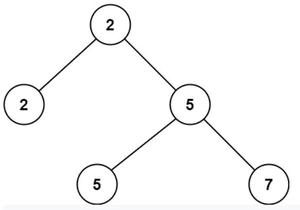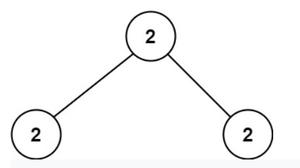# Second unique smallest value of given Binary Tree whose each node is minimum of its children

• Last Updated : 28 Jun, 2022

Given a full binary tree where each node value is the same as the minimum value between its children, the task is to find the second minimum unique value of the tree.

Examples:

Input: tree:Example of the problem statement

Output: 5
Explanation: All the unique values present in the tree are 2, 5 and 7.
The second smallest is 5.

Input: tree:Another example of a problem statement

Output: -1
Explanation: All the numbers present in the tree are same.
There is no other value except 2, so second smallest is not possible.

Naive Approach: The basic approach to solve the problem is based on the idea:

Store all the unique values in an array and sort the array. Then find the second minimum from the array.

Follow the below step to understand the approach more clearly:

• Traverse the tree and store all the values in an array.
• Sort the array.
• Iterate over the array and find the first array element which is not equal to the minimum element of the array (i.e. the one present at index 0). If no such element is present then return -1.

Below is the implementation of the above approach.

## C++

 `// C++ code to implement the above approach` `#include ``using` `namespace` `std;` `// Structure of a tree node``struct` `Node {``    ``int` `data;``    ``struct` `Node* left;``    ``struct` `Node* right;``    ``Node(``int` `val)``    ``{``        ``data = val;``        ``left = NULL;``        ``right = NULL;``    ``}``};` `// Initialize a vector``vector<``int``> ans;` `// Traversing the tree by using inorder``// traversal``void` `inorderTraversal(Node* root)``{``    ``if` `(root != NULL) {``        ``inorderTraversal(root->left);``        ``ans.push_back(root->data);``        ``inorderTraversal(root->right);``    ``}``}` `// Function to find the second minimum element``int` `findSecondMinimumValue(Node* root)``{``    ``inorderTraversal(root);` `    ``// Sorting the array element``    ``sort(ans.begin(), ans.end());` `    ``// Traversing and array and checking``    ``// the second minimum element``    ``for` `(``int` `i = 0; i < ans.size() - 1; i++)``        ``if` `(ans[i] != ans[i + 1])``            ``return` `ans[i + 1];``    ``return` `-1;``}` `// Driver code``int` `main()``{``    ``// Creating the tree``    ``// 2``    ``//        / \``    ``// 2   5``    ``//          / \``    ``// 5   7``    ``struct` `Node* root = ``new` `Node(2);``    ``root->left = ``new` `Node(2);``    ``root->right = ``new` `Node(5);``    ``root->right->left = ``new` `Node(5);``    ``root->right->right = ``new` `Node(7);` `    ``// Function call``    ``int` `SecondMinimumValue``        ``= findSecondMinimumValue(root);``    ``cout << SecondMinimumValue << endl;``    ``return` `0;``}`

## Java

 `// JAVA code to implement the above approach``import` `java.util.*;` `class` `GFG``{` `  ``// Structure of a tree node``  ``public` `static` `class` `Node {``    ``int` `data;``    ``Node left;``    ``Node right;``    ``Node(``int` `val) { ``this``.data = val; }``  ``}` `  ``// Initialize a vector``  ``public` `static` `ArrayList ans``    ``= ``new` `ArrayList();` `  ``// Traversing the tree by using inorder``  ``// traversal``  ``public` `static` `void` `inorderTraversal(Node root)``  ``{``    ``if` `(root != ``null``) {``      ``inorderTraversal(root.left);``      ``ans.add(root.data);``      ``inorderTraversal(root.right);``    ``}``  ``}` `  ``// Function to find the second minimum element``  ``public` `static` `int` `findSecondMinimumValue(Node root)``  ``{``    ``inorderTraversal(root);` `    ``// Sorting the array element``    ``Collections.sort(ans);` `    ``// Traversing and array and checking``    ``// the second minimum element``    ``for` `(``int` `i = ``0``; i < ans.size() - ``1``; i++)``      ``if` `(ans.get(i) != ans.get(i + ``1``))``        ``return` `ans.get(i + ``1``);``    ``return` `-``1``;``  ``}` `  ``// Driver code``  ``public` `static` `void` `main(String[] args)``  ``{``    ``// Creating the tree``    ``// 2``    ``//        / \``    ``// 2   5``    ``//          / \``    ``// 5   7``    ``Node root = ``new` `Node(``2``);``    ``root.left = ``new` `Node(``2``);``    ``root.right = ``new` `Node(``5``);``    ``root.right.left = ``new` `Node(``5``);``    ``root.right.right = ``new` `Node(``7``);` `    ``// Function call``    ``int` `SecondMinimumValue``      ``= findSecondMinimumValue(root);``    ``System.out.println(SecondMinimumValue);``  ``}``}` `// This code is contributed by Taranpreet`

## Python3

 `# Python3 code for the above approach` `# class to create a tree node``class` `Node:``    ``def` `__init__(``self``, d):``        ``self``.data ``=` `d``        ``self``.left ``=` `None``        ``self``.right ``=` `None``            ` `# Initialize a list``ans ``=` `[]` `# Traversing the tree by using inorder``# traversal``def` `inorderTraversal(root) :``    ``if` `(root !``=` `None``) :``        ``inorderTraversal(root.left)``        ``ans.append(root.data)``        ``inorderTraversal(root.right)``            ` `        `  `    ``# Function to find the second minimum element``def` `findSecondMinimumValue(root) :``    ``inorderTraversal(root)` `    ``# Sorting the array element``    ``ans.sort()` `    ``# Traversing and array and checking``    ``# the second minimum element``    ``for` `i ``in` `range``(``0``,``len``(ans)``-``1``) :``        ``if` `(ans[i] !``=` `ans[i ``+` `1``]) :``            ``return` `ans[i ``+` `1``]``    ``return` `-``1` `# Driver Code``if` `__name__ ``=``=` `'__main__'``:``        ` `    ``# Driver code` `    ``# Creating the tree``    ``#   2``    ``#  / \``    ``# 2   5``    ``#    / \``    ``#   5   7``    ``root ``=` `Node(``2``)``    ``root.left ``=` `Node(``2``)``    ``root.right ``=` `Node(``5``)``    ``root.right.left ``=` `Node(``5``)``    ``root.right.right ``=` `Node(``7``)` `    ``# Function call``    ``SecondMinimumValue ``=` `findSecondMinimumValue(root)``    ``print``(SecondMinimumValue)` `    ``# This code is contributed by jana_sayantan.`

## Javascript

 ``

## C#

 `// C# code to implement the above approach``using` `System;``using` `System.Collections.Generic;` `public` `class` `GFG``{` `  ``// Structure of a tree node``  ``public` `class` `Node {``    ``public` `int` `data;``    ``public` `Node left;``    ``public` `Node right;``    ``public` `Node(``int` `val) { ``this``.data = val; }``  ``}` `  ``// Initialize a vector``  ``public` `static` `List<``int``> ans``    ``= ``new` `List<``int``>();` `  ``// Traversing the tree by using inorder``  ``// traversal``  ``public` `static` `void` `inorderTraversal(Node root)``  ``{``    ``if` `(root != ``null``) {``      ``inorderTraversal(root.left);``      ``ans.Add(root.data);``      ``inorderTraversal(root.right);``    ``}``  ``}` `  ``// Function to find the second minimum element``  ``public` `static` `int` `findSecondMinimumValue(Node root)``  ``{``    ``inorderTraversal(root);` `    ``// Sorting the array element``    ``ans.Sort();` `    ``// Traversing and array and checking``    ``// the second minimum element``    ``for` `(``int` `i = 0; i < ans.Count - 1; i++)``      ``if` `(ans[i] != ans[i + 1])``        ``return` `ans[i + 1];``    ``return` `-1;``  ``}` `  ``// Driver code``  ``public` `static` `void` `Main(String[] args)``  ``{``    ``// Creating the tree``    ``// 2``    ``//        / \``    ``// 2   5``    ``//          / \``    ``// 5   7``    ``Node root = ``new` `Node(2);``    ``root.left = ``new` `Node(2);``    ``root.right = ``new` `Node(5);``    ``root.right.left = ``new` `Node(5);``    ``root.right.right = ``new` `Node(7);` `    ``// Function call``    ``int` `SecondMinimumValue``      ``= findSecondMinimumValue(root);``    ``Console.WriteLine(SecondMinimumValue);``  ``}``}`  `// This code contributed by shikhasingrajput`

Output

`5`

Time Complexity: O(N * logN)
Auxiliary Space: O(N)

Efficient Approach: The problem can be solved efficiently by using the below observation:

The root node of the tree contains the minimum among all the nodes.
If the value of all the nodes with minimum value is replaced by some other arbitrary numbers out of the range of tree values and the tree property is maintained then the root node will have the current minimum value which is actually the second minimum of the given tree.

The above observation can be implemented using recursion. Follow the approach mentioned below to implement the idea of the above observation:

• Use a recursive function to traverse the tree and implement the observation.
• In each recursion for any node:
• Find which of its children has the same value as the root and call the next recursion for that child.
• If the value of the current node is the same as the root and does not have any children, or both children have same value replace its value with -1.
• If the current node has children, replace it with the minimum of its children while returning from the recursive function. (This will replace the value with the second minimum as the minimum is replaced with -1 and -1 is not being considered for checking the minimum).
• In this way, while the traversal is complete, the tree root holds the current minimum of the tree which is actually the second minimum of the original tree.
• Return the value of the root.

Below is the code of the above approach:

## C++

 `// C++ program for above approach` `#include ``using` `namespace` `std;` `// Structure of a tree node``struct` `Node {``    ``int` `data;``    ``struct` `Node* left;``    ``struct` `Node* right;``    ``Node(``int` `val)``    ``{``        ``data = val;``        ``left = NULL;``        ``right = NULL;``    ``}``};` `// Function to find the minimum value``int` `findSecondMinimumValue(Node* root)``{``    ``// When the root is null``    ``if` `(!root)``        ``return` `-1;` `    ``// Base Condition``    ``// When we reach the Leaf node then``    ``// in that case we have to return -1``    ``// as per the algorithm stated in``    ``// the above statement``    ``if` `(!root->left && !root->right)``        ``return` `-1;` `    ``// Storing the Node value of the left``    ``// child of the Node``    ``auto` `left = root->left->data;` `    ``// Storing the Node value of the right``    ``// child of the Node``    ``auto` `right = root->right->data;` `    ``// Call the function recursively to the``    ``// left sub-part of the tree if the value``    ``// of the node value matches with its left``    ``// child node value``    ``if` `(root->data == root->left->data)``        ``left``            ``= findSecondMinimumValue(root->left);` `    ``// Call the function recursively to the``    ``// right sub-part of the tree if the``    ``// value of the node value matches with``    ``// its right child node value``    ``if` `(root->data == root->right->data)``        ``right``            ``= findSecondMinimumValue(root->right);` `    ``// In case if both the left and right``    ``// child value is not equal to -1 then``    ``// in that case return the minimum of``    ``// them to the its parent``    ``if` `(left != -1 && right != -1)``        ``return` `min(left, right);` `    ``// In case if the left child's value is``    ``// not equal to -1 BUT its right child's``    ``// value is equal to -1 then in that case``    ``// send the left child value to its``    ``// parent node.``    ``else` `if` `(left != -1)``        ``return` `left;` `    ``// In case if the right child's value is``    ``// not equal to -1 BUT its left child's``    ``// value is equal to -1 then in that case``    ``// send the right child value to its``    ``// parent node.``    ``else``        ``return` `right;``}` `// Driver code``int` `main()``{``    ``// Creating the root node``    ``/*         2``              ``/ \``             ``2   5``                ``/ \``               ``5   7 */``    ``struct` `Node* root = ``new` `Node(2);``    ``root->left = ``new` `Node(2);``    ``root->right = ``new` `Node(5);``    ``root->right->left = ``new` `Node(5);``    ``root->right->right = ``new` `Node(7);` `    ``int` `SecondMinimumValue``        ``= findSecondMinimumValue(root);``    ``cout << SecondMinimumValue << endl;``    ``return` `0;``}`

## C

 `// C program for above approach` `#include ``#include ` `// Structure of a tree node``typedef` `struct` `Node {``    ``int` `data;``    ``struct` `Node* left;``    ``struct` `Node* right;``} Node;` `Node* newNode(``int` `data)``{``    ``Node* new_node = (Node*)``malloc``(``sizeof``(Node));``    ``new_node->data = data;``    ``new_node->left = new_node->right = NULL;``    ``return` `new_node;``}` `// Find minimum between two numbers.``int` `min(``int` `num1, ``int` `num2)``{``  ``return` `(num1 > num2) ? num2 : num1;``}` `// Function to find the minimum value``int` `findSecondMinimumValue(Node* root)``{``    ``// When the root is null``    ``if` `(!root)``        ``return` `-1;` `    ``// Base Condition``    ``// When we reach the Leaf node then``    ``// in that case we have to return -1``    ``// as per the algorithm stated in``    ``// the above statement``    ``if` `(!root->left && !root->right)``        ``return` `-1;` `    ``// Storing the Node value of the left``    ``// child of the Node``    ``int` `left = root->left->data;` `    ``// Storing the Node value of the right``    ``// child of the Node``    ``int` `right = root->right->data;` `    ``// Call the function recursively to the``    ``// left sub-part of the tree if the value``    ``// of the node value matches with its left``    ``// child node value``    ``if` `(root->data == root->left->data)``        ``left = findSecondMinimumValue(root->left);` `    ``// Call the function recursively to the``    ``// right sub-part of the tree if the``    ``// value of the node value matches with``    ``// its right child node value``    ``if` `(root->data == root->right->data)``        ``right = findSecondMinimumValue(root->right);` `    ``// In case if both the left and right``    ``// child value is not equal to -1 then``    ``// in that case return the minimum of``    ``// them to the its parent``    ``if` `(left != -1 && right != -1)``        ``return` `min(left, right);` `    ``// In case if the left child's value is``    ``// not equal to -1 BUT its right child's``    ``// value is equal to -1 then in that case``    ``// send the left child value to its``    ``// parent node.``    ``else` `if` `(left != -1)``        ``return` `left;` `    ``// In case if the right child's value is``    ``// not equal to -1 BUT its left child's``    ``// value is equal to -1 then in that case``    ``// send the right child value to its``    ``// parent node.``    ``else``        ``return` `right;``}` `// Driver code``int` `main()``{``    ``// Creating the root node``    ``/*         2``              ``/ \``             ``2   5``                ``/ \``               ``5   7 */``    ``Node* root = newNode(2);``    ``root->left = newNode(2);``    ``root->right = newNode(5);``    ``root->right->left = newNode(5);``    ``root->right->right = newNode(7);` `    ``int` `SecondMinimumValue = findSecondMinimumValue(root);``    ``printf``(``"%d\n"``, SecondMinimumValue);``    ``return` `0;``}` `// This code is contributed by Sania Kumari Gupta`

## Python3

 `# Python program to implement above approach` `# Structure of a tree node``class` `Node:``    ``def` `__init__(``self``,val):``        ``self``.data ``=` `val``        ``self``.left ``=` `None``        ``self``.right ``=` `None``    `  `# Function to find the minimum value``def` `findSecondMinimumValue(root):``    ``# When the root is None``    ``if` `(root ``=``=` `None``):``        ``return` `-``1` `    ``# Base Condition``    ``# When we reach the Leaf node then``    ``# in that case we have to return -1``    ``# as per the algorithm stated in``    ``# the above statement``    ``if` `(root.left ``=``=` `None` `and` `root.right ``=``=` `None``):``        ``return` `-``1` `    ``# Storing the Node value of the left``    ``# child of the Node``    ``left ``=` `root.left.data` `    ``# Storing the Node value of the right``    ``# child of the Node``    ``right ``=` `root.right.data` `    ``# Call the function recursively to the``    ``# left sub-part of the tree if the value``    ``# of the node value matches with its left``    ``# child node value``    ``if` `(root.data ``=``=` `root.left.data):``        ``left ``=` `findSecondMinimumValue(root.left)` `    ``# Call the function recursively to the``    ``# right sub-part of the tree if the``    ``# value of the node value matches with``    ``# its right child node value``    ``if` `(root.data ``=``=` `root.right.data):``        ``right ``=` `findSecondMinimumValue(root.right)` `    ``# In case if both the left and right``    ``# child value is not equal to -1 then``    ``# in that case return the minimum of``    ``# them to the its parent``    ``if` `(left !``=` `-``1` `and` `right !``=` `-``1``):``        ``return` `min``(left, right)` `    ``# In case if the left child's value is``    ``# not equal to -1 BUT its right child's``    ``# value is equal to -1 then in that case``    ``# send the left child value to its``    ``# parent node.``    ``elif` `(left !``=` `-``1``):``        ``return` `left` `    ``# In case if the right child's value is``    ``# not equal to -1 BUT its left child's``    ``# value is equal to -1 then in that case``    ``# send the right child value to its``    ``# parent node.``    ``else``:``        ``return` `right` `# Driver code` `root ``=` `Node(``2``)``root.left ``=` `Node(``2``)``root.right ``=` `Node(``5``)``root.right.left ``=` `Node(``5``)``root.right.right ``=` `Node(``7``)``        ` `SecondMinimumValue ``=` `findSecondMinimumValue(root)``print``(SecondMinimumValue)` `# This code is contributed by shinjanpatra`

## Javascript

 ``

Output

`5`

Time Complexity: O(H) where H is the height of the tree
Auxiliary Space: O(1)

My Personal Notes arrow_drop_up